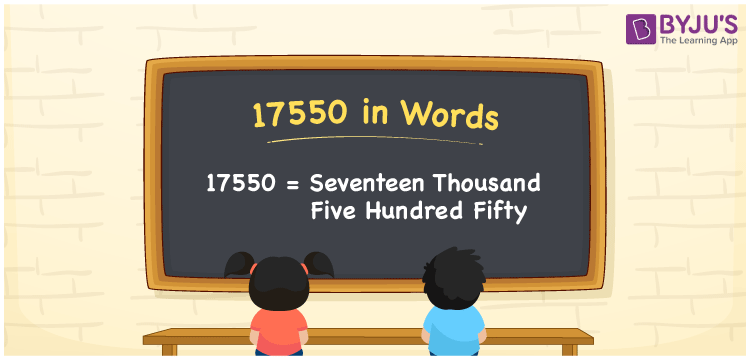# 17550 in Words

The numeral 17550 in words is written as “seventeen thousand five hundred fifty”. The natural number 17550 is a five-digit number and its number name can be written using the place value system. Also, 17550 is a cardinal number. For example, the cost of 10 books is Rs. 17550.

 17550 in Words: Seventeen Thousand Five Hundred Fifty. Seventeen Thousand Five Hundred Fifty in Numerical Form: 17550.

## 17550 in English Words## How to Write 17550 in Words?

The following table depicts the place values of the number 17550.

 Ten-thousands Thousands Hundreds Tens Ones 1 7 5 5 0

The expanded form of 17550 is as follows:

= 1 × Ten thousand + 7 × Thousand + 5 × Hundred + 5 × Ten + 0 × One

= 1 × 10000 + 7 × 1000 + 5 × 100 + 5 × 10 + 0 × 1

= 10000 + 7000 + 500 + 50

= 17550

= Seventeen thousand five hundred fifty

Hence, 17550 in words is seventeen thousand five hundred fifty.

17550 in words – Seventeen thousand five hundred fifty

Is 17550 an odd number? – No

Is 17550 an even number? – Yes

Is 17550 a perfect square number? – No

Is 17550 a perfect cube number? – No

Is 17550 a prime number? – No

Is 17550 a composite number? – Yes

## Frequently Asked Questions on 17550 in Words

Q1

### How to spell 17550 in words?

17550 in words is seventeen thousand five hundred fifty.

Q2

### Simplify 17000 + 550, and express it in words.

Simplifying 17000 + 550, we get 17550. Hence, 17550 in words is seventeen thousand five hundred fifty.

Q3

### Is 17550 a composite number?

Yes, 17550 is a composite number.